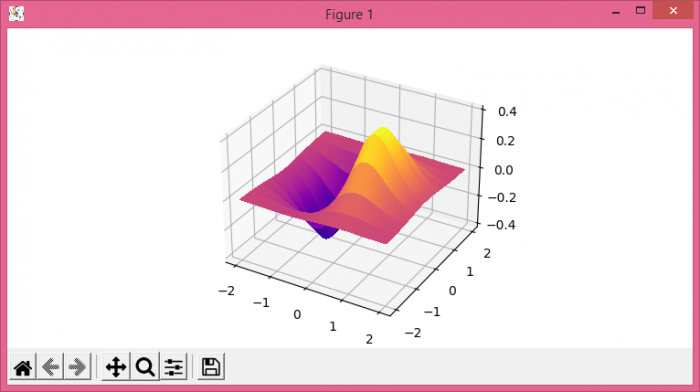# Plot a 3D surface from {x,y,z}-scatter data in Python Matplotlib

To plot a 3D surface from x, y and z scatter data in Python, we can take the following steps −

• Set the figure size and adjust the padding between and around the subplots.
• Create a new figure or activate an existing figure using figure() method.
• Add an axes to the figure as part of a subplot arrangement.
• Create x, y, X, Y and Z data points using numpy.
• Plot x, y and z data points using plot_surface() method.
• To display the figure, use show() method.

## Example

import matplotlib.pyplot as plt
import numpy as np

plt.rcParams["figure.figsize"] = [7.50, 3.50]
plt.rcParams["figure.autolayout"] = True

fig = plt.figure()

x = np.array(np.linspace(-2, 2, 100))
y = np.array(np.linspace(-2, 2, 10))

X, Y = np.meshgrid(x, y)
Z = X * np.exp(-X ** 2 - Y ** 2)

ax.plot_surface(X, Y, Z, rstride=1, cstride=1, cmap="plasma", linewidth=0, antialiased=False)

plt.show()

## Output